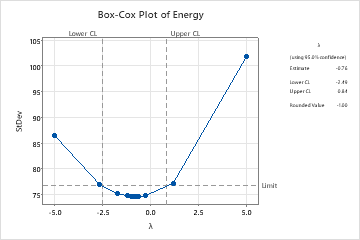# Example of Box-Cox Transformation

A textile manufacturer developed a solar energy system to pre-heat feed water for a boiler that is part of the power system for the manufacturing process. A technician monitors the amount of energy that is used each hour to make sure that the pre-heat process is stable.

Before creating an I-MR chart of the data, the technician conducts a normality test on the data and finds that the data do not follow a normal distribution. The technician then conducts a Box-Cox analysis to determine whether a Box-Cox transformation is appropriate.

1. Open the sample data, SolarEnergyProcess.MTW.
2. Choose Stat > Control Charts > Box-Cox Transformation.
3. In All observations for a chart are in one column, enter Energy.
4. In Subgroup sizes, enter 1.
5. Click OK.

## Interpret the results

For the Box-Cox transformation, a λ value of 1 is equivalent to using the original data. Therefore, if the confidence interval for the optimal λ includes 1, then no transformation is necessary. In this example, the 95% confidence interval for λ (−2.87 to 0.66) does not include 1, so a transformation is appropriate. The estimated value for the optimal λ is −1.03. Because the rounded value of −1 is within the confidence interval, the technician should transform the data using λ = −1. A transformation that uses λ = −1 corresponds to the inverse transformation (transformed value = 1 / original value).By using this site you agree to the use of cookies for analytics and personalized content.  Read our policy